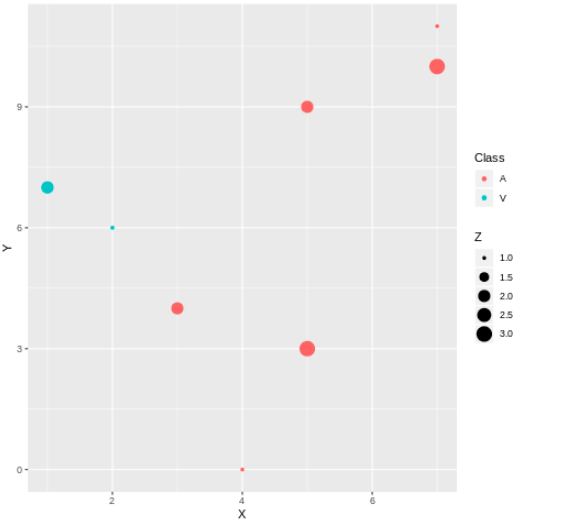# 在数据科学中你需要尝试的10个有用的Python技巧

### 概览

• 在数据科学中10个有用而创新的Python技巧
• 这个Python技巧列表包含我们日常数据科学任务的用例，例如数据预处理，数据分析，可视化等等
• 来看一下之前我们错过的Python包或函数吧

### 介绍

Python不仅限于Pandas，NumPy和scikit-learn(尽管它们在数据科学中绝对必不可少)！我们可以使用很多Python技巧来改进我们的代码，加速我们的数据科学任务，并在编写代码时变得更加高效。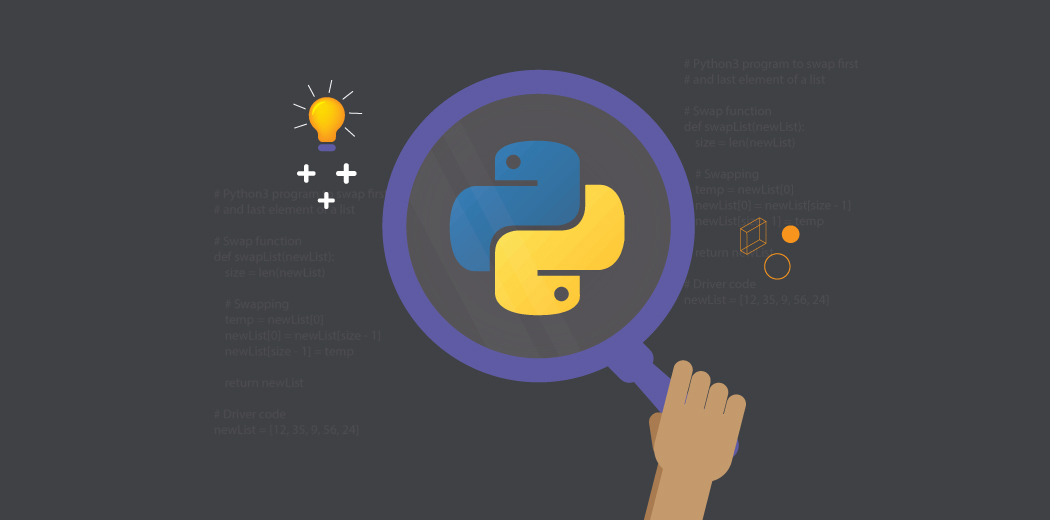### 1. zip: 在Python中组合多个列表

[code lang=python]
a = [1,2,3]
b = [4,5,6]
c = [4,5,6,7,8]
zipped = zip(a,b) # 打包为元组的列表
for ele in zipped:
print(ele,end="\t")
print()

zipped = zip(a,c) # 元素个数与最短的列表一致
for ele in zipped:
print(ele,end="\t")
[/code]#### 安装gmplot

[code lang=text]
!pip3 install gmplot
[/code]

[code lang=python]
import pandas as pd
import gmplot
[/code]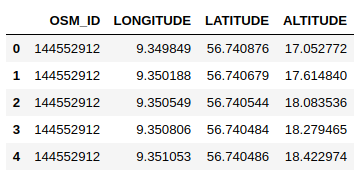[code lang=python]
# 纬度和经度列表
latitude_list = data['LATITUDE']
longitude_list = data['LONGITUDE']

# 地图的中心坐标

# 在谷歌地图上绘制坐标
gmap.scatter( latitude_list, longitude_list, '# FF0000', size = 40, marker = True)

#以下代码将在你的Web浏览器中创建html文件视图
gmap.heatmap(latitude_list, longitude_list)

gmap.draw( "mymap.html" )
[/code]

### 3. category_encoders: 使用15种不同的编码方案对你的类别变量进行编码

#### 安装category-encoders

[code lang=text]
!pip3 install category-encoders
[/code]

#### 将类别数据转换为数值数据

[code lang=python]
import pandas as pd
import category_encoders as ce

# 创建一个Dataframe
data = pd.DataFrame({ 'gender' : ['Male', 'Female', 'Male', 'Female', 'Female'],
'class' : ['A','B','C','D','A'],
'city' : ['Delhi','Gurugram','Delhi','Delhi','Gurugram'] })
[/code]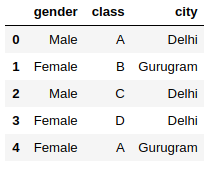[code lang=python]
# 热编码
# 创建One Hot Encoder对象

ce_OHE = ce.OneHotEncoder(cols=['gender','city'])

# 转换数据
data = ce_OHE.fit_transform(data)
[/code]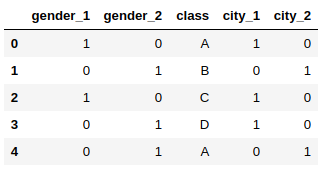### 4. progress_apply:监控你在数据科学任务上花费的时间

[code lang=python]
import pandas as pd
from tqdm._tqdm_notebook import tqdm_notebook
tqdm_notebook.pandas()
[/code][code lang=python]
def calculate_sum(x):
return (x['LATITUDE']+x['LONGITUDE'])

data['DISTANCE'] = data.progress_apply(calculate_distance,axis=1)
[/code]### 5.pandas_profiling:生成数据集的详细报告

pandas_profiling是一个Python包，可以减少执行最初数据分析步骤的大量工作。该软件包只需一行代码即可生成详细的数据报告！

[code lang=python]
import pandas as pd
import pandas_profiling

# 读取数据
pfr = pandas_profiling.ProfileReport(data)
# 在jupyter执行代码,则不需下面一行代码
pfr.to_file("./example.html")
[/code]

– 警告
– 所有类别变量的频率计数
– 数值变量的分位数和描述性统计
– 相关图

### 6. grouper: 分组时间序列数据

[code lang=python]
import pandas as pd

[/code]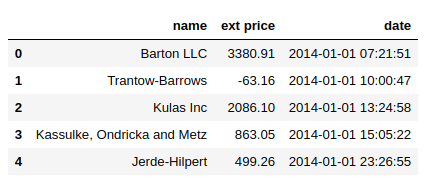[code lang=python]
data['date'] = pd.to_datetime(data['date'])
[/code]

[code lang=python]
data.set_index('date').groupby('name')["ext price"].resample("M").sum()
[/code]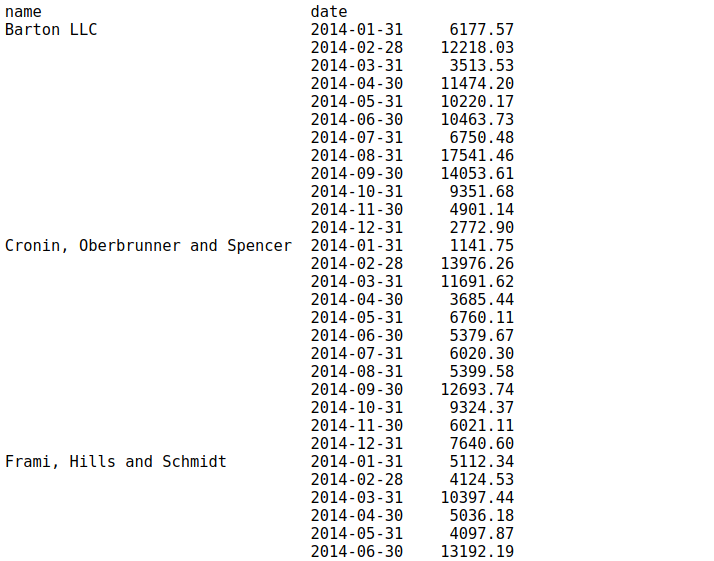[code lang=python]
data.groupby(['name', pd.Grouper(key='date', freq='M')])['ext price'].sum()
[/code]### 7. unstack: 将索引转换为Dataframe的列

[code lang=python]
data.groupby(['name', pd.Grouper(key='date', freq='M')])['ext price'].sum().unstack()
[/code]### 8. %matplotlib Notebook: Jupyter Notebook中的交互式图

[code lang=python]
%matplotlib notebook
import matplotlib.pyplot as plt

# 绘制散点图
#在你的数据集上尝试此操作
plt.scatter(data['quantity'],data['unit price'])
[/code]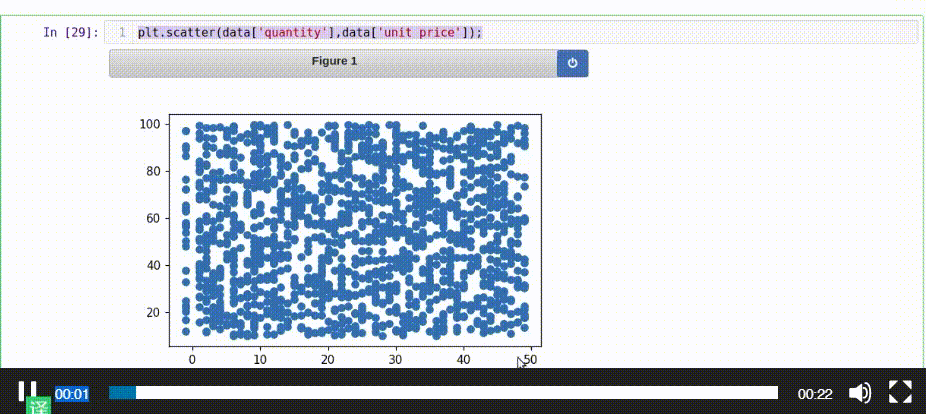### 9. %%time: 检查特定Python代码块的运行时间

[code lang=python]
%%time
def myfunction(x) :
for i in range(1,100000,1) :
i=i+1
myfunction(3)
[/code]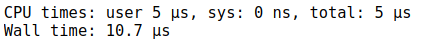### 10: rpy2: R和Python在同一个Jupyter Notebook中！

R和Python是数据科学领域中最好和最流行的两种开源编程语言。R主要用于统计分析，而Python提供了一个简单的接口，可以将数学解决方案转换为代码。

[code lang=text]
!pip3 install rpy2
[/code]

[code lang=python]
%R require(ggplot2)
[/code]

[code lang=python]
import pandas as pd
df = pd.DataFrame({
'Class': ['A', 'A', 'A', 'V', 'V', 'A', 'A', 'A'],
'X': [4, 3, 5, 2, 1, 7, 7, 5],
'Y': [0, 4, 3, 6, 7, 10, 11, 9],
'Z': [1, 2, 3, 1, 2, 3, 1, 2]
})
[/code]

[code lang=python]
%%R -i df
ggplot(data = df) + geom_point(aes(x = X, y= Y, color = Class, size = Z))
[/code]